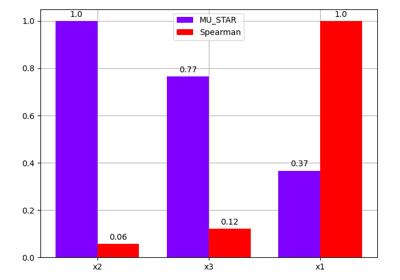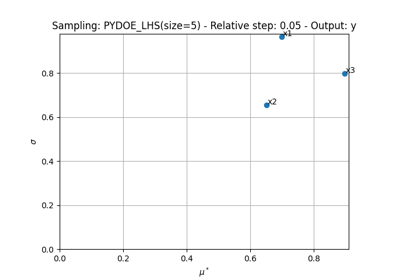gemseo / uncertainty / sensitivity / morris

# analysis module¶

Class for the estimation of Morris indices.

## OAT technique¶

The purpose of the One-At-a-Time (OAT) methodology is to quantify the elementary effect

$df_i = f(X_1+dX_1,\ldots,X_{i-1}+dX_{i-1},X_i+dX_i,\ldots,X_d) - f(X_1+dX_1,\ldots,X_{i-1}+dX_{i-1},X_i,\ldots,X_d)$

associated with a small variation $$dX_i$$ of $$X_i$$ with

$df_1 = f(X_1+dX_1,\ldots,X_d)-f(X_1,\ldots,X_d)$

The elementary effects $$df_1,\ldots,df_d$$ are computed sequentially from an initial point

$X=(X_1,\ldots,X_d)$

From these elementary effects, we can compare their absolute values $$|df_1|,\ldots,|df_d|$$ and sort $$X_1,\ldots,X_d$$ accordingly.

## Morris technique¶

Then, the purpose of the Morris’ methodology is to repeat the OAT method from different initial points $$X^{(1)},\ldots,X^{(r)}$$ and compare the parameters in terms of mean

$\mu_i^* = \frac{1}{r}\sum_{j=1}^r|df_i^{(j)}|$

and standard deviation

$\sigma_i = \frac{1}{r}\sum_{j=1}^r\left(|df_i^{(j)}|-\mu_i\right)^2$

where $$\mu_i = \frac{1}{r}\sum_{j=1}^rdf_i^{(j)}$$.

This methodology relies on the MorrisAnalysis class.

class gemseo.uncertainty.sensitivity.morris.analysis.MorrisAnalysis(disciplines, parameter_space, n_samples, output_names=(), algo='', algo_options=mappingproxy({}), n_replicates=5, step=0.05, formulation='MDF', **formulation_options)[source]

Sensitivity analysis based on the Morris’ indices.

MorrisAnalysis.indices contains both $$\mu^*$$, $$\mu$$ and $$\sigma$$ while MorrisAnalysis.main_indices represents $$\mu^*$$. Lastly, the MorrisAnalysis.plot() method represents the parameters as a scatter plot where $$X_i$$ has as coordinates $$(\mu_i^*,\sigma_i)$$. The bigger $$\mu_i^*$$ is, the more significant $$X_i$$ is. Concerning $$\sigma_i$$, it highlights non-linear effects along $$X_i$$ or cross-effects between $$X_i$$ and other parameter(s).

The user can specify the DOE algorithm name to select the initial points, as well as the number of replicates and the relative step for the input variations.

Examples

>>> from numpy import pi
>>> from gemseo import create_discipline, create_parameter_space
>>> from gemseo.uncertainty.sensitivity.morris.analysis import MorrisAnalysis
>>>
>>> expressions = {"y": "sin(x1)+7*sin(x2)**2+0.1*x3**4*sin(x1)"}
>>> discipline = create_discipline(
...     "AnalyticDiscipline", expressions=expressions
... )
>>>
>>> parameter_space = create_parameter_space()
...     "x1", "OTUniformDistribution", minimum=-pi, maximum=pi
... )
...     "x2", "OTUniformDistribution", minimum=-pi, maximum=pi
... )
...     "x3", "OTUniformDistribution", minimum=-pi, maximum=pi
... )
>>>
>>> analysis = MorrisAnalysis([discipline], parameter_space, n_samples=None)
>>> indices = analysis.compute_indices()

Parameters:
• disciplines (Collection[MDODiscipline]) – The discipline or disciplines to use for the analysis.

• parameter_space (ParameterSpace) – A parameter space.

• n_samples (int | None) – A number of samples. If None, the number of samples is computed by the algorithm.

• output_names (Iterable[str]) –

The disciplines’ outputs to be considered for the analysis. If empty, use all the outputs.

By default it is set to ().

• algo (str) –

The name of the DOE algorithm. If empty, use the SensitivityAnalysis.DEFAULT_DRIVER.

By default it is set to “”.

• algo_options (Mapping[str, DOELibraryOptionType]) –

The options of the DOE algorithm.

By default it is set to {}.

• n_replicates (int) –

The number of times the OAT method is repeated. Used only if n_samples is None. Otherwise, this number is the greater integer $$r$$ such that $$r(d+1)\leq$$ n_samples and $$r(d+1)$$ is the number of samples actually carried out.

By default it is set to 5.

• step (float) –

The finite difference step of the OAT method.

By default it is set to 0.05.

• formulation (str) –

The name of the MDOFormulation to sample the disciplines.

By default it is set to “MDF”.

• **formulation_options (Any) – The options of the MDOFormulation.

Raises:

ValueError – If at least one input dimension is not equal to 1.

class Method(value)[source]

Bases: StrEnum

The names of the sensitivity methods.

MU_STAR = 'MU_STAR'

The mean of the absolute finite difference.

SIGMA = 'SIGMA'

The standard deviation of the absolute finite difference.

compute_indices(outputs=(), normalize=False)[source]

Compute the sensitivity indices.

Parameters:
• outputs (str | Sequence[str]) –

The output(s) for which to display the sensitivity indices. If None, use the default outputs set at instantiation.

By default it is set to ().

• normalize (bool) –

Whether to normalize the indices with the empirical bounds of the outputs.

By default it is set to False.

Returns:

The sensitivity indices.

With the following structure:

{
"method_name": {
"output_name": [
{
"input_name": data_array,
}
]
}
}


Return type:

dict[str, FirstOrderIndicesType]

plot(output, inputs=(), title='', save=True, show=False, file_path='', directory_path='', file_name='', file_format='', offset=1, lower_mu=None, lower_sigma=None)[source]

Plot the Morris indices for each input variable.

For $$i\in\{1,\ldots,d\}$$, plot $$\mu_i^*$$ in function of $$\sigma_i$$.

Parameters:
• output (VariableType) – The output for which to display sensitivity indices, either a name or a tuple of the form (name, component). If name, its first component is considered.

• inputs (Iterable[str]) –

The uncertain input variables for which to display the sensitivity indices. If empty, display all the uncertain input variables.

By default it is set to ().

• title (str) –

The title of the plot, if any.

By default it is set to “”.

• save (bool) –

If True, save the figure.

By default it is set to True.

• show (bool) –

If True, show the figure.

By default it is set to False.

• file_path (str | Path) –

A file path. Either a complete file path, a directory name or a file name. If empty, use a default file name and a default directory. The file extension is inferred from filepath extension, if any.

By default it is set to “”.

• directory_path (str | Path) –

The description is missing.

By default it is set to “”.

• file_name (str) –

The description is missing.

By default it is set to “”.

• file_format (str) –

A file format, e.g. ‘png’, ‘pdf’, ‘svg’, … Used when file_path does not have any extension. If empty, use a default file extension.

By default it is set to “”.

• offset (float) –

The offset to display the inputs names, expressed as a percentage applied to both x-range and y-range.

By default it is set to 1.

• lower_mu (float | None) – The lower bound for $$\mu$$. If None, use a default value.

• lower_sigma (float | None) – The lower bound for $$\sigma$$. If None, use a default value.

Return type:

None

DEFAULT_DRIVER = 'lhs'
dataset: IODataset

The dataset containing the discipline evaluations.

default_output: Iterable[str]

The default outputs of interest.

property indices: dict[str, Dict[str, List[Dict[str, numpy.ndarray]]]]

The sensitivity indices.

With the following structure:

{
"method_name": {
"output_name": [
{
"input_name": data_array,
}
]
}
}

max: dict[str, dict[str, ndarray]]

The maximum effect with the following structure:

{
"output_name": [
{
"input_name": data_array,
}
]
}

min: dict[str, dict[str, ndarray]]

The minimum effect with the following structure:

{
"output_name": [
{
"input_name": data_array,
}
]
}

mu_: dict[str, dict[str, ndarray]]

The mean effects with the following structure:

{
"output_name": [
{
"input_name": data_array,
}
]
}

mu_star: dict[str, dict[str, ndarray]]

The mean absolute effects with the following structure:

{
"output_name": [
{
"input_name": data_array,
}
]
}

property n_replicates: int

The number of OAT replicates.

property outputs_bounds: dict[str, list[float]]

The empirical bounds of the outputs.

relative_sigma: dict[str, dict[str, ndarray]]

The relative variability of the effects with the following structure:

{
"output_name": [
{
"input_name": data_array,
}
]
}

sigma: dict[str, dict[str, ndarray]]

The variability of the effects with the following structure:

{
"output_name": [
{
"input_name": data_array,
}
]
}


## Examples using MorrisAnalysis¶Comparing sensitivity indices

Comparing sensitivity indicesMorris analysis

Morris analysis